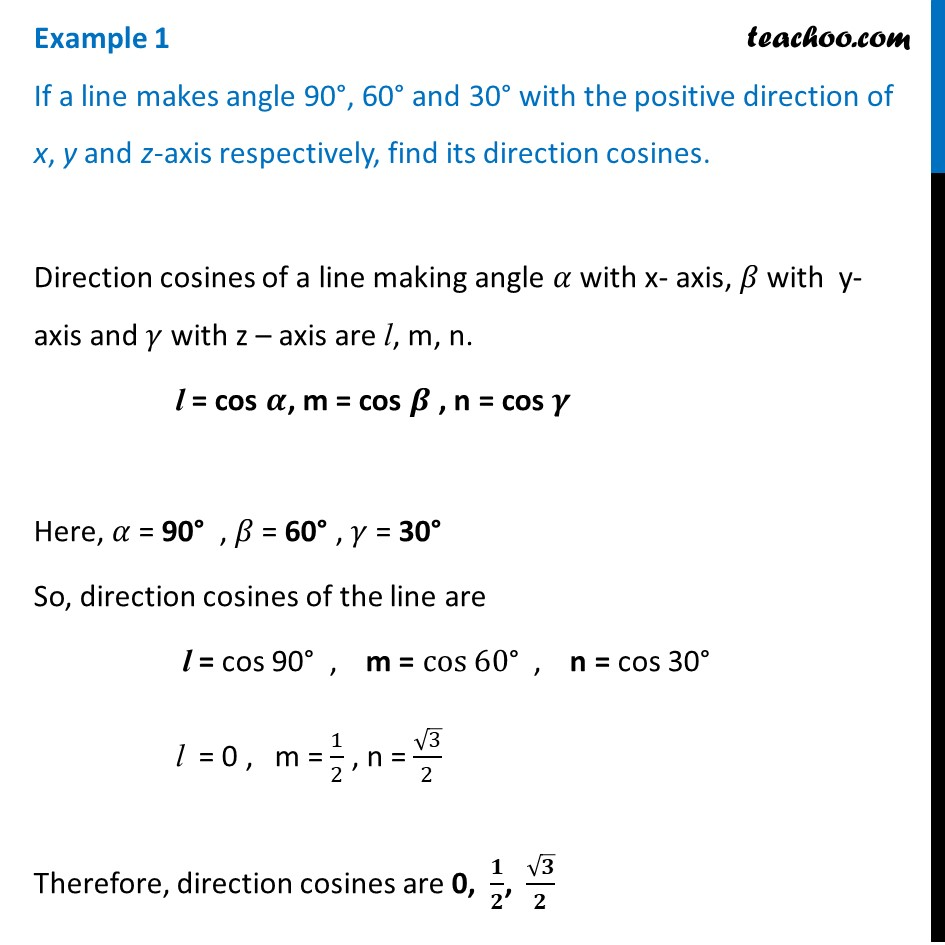Direction cosines and ratios

Chapter 11 Class 12 Three Dimensional Geometry
Concept wiseLearn in your speed, with individual attention - Teachoo Maths 1-on-1 Class

### Transcript

Example 1 If a line makes angle 90°, 60° and 30° with the positive direction of x, y and z-axis respectively, find its direction cosines.Direction cosines of a line making angle 𝛼 with x- axis, 𝛽 with y- axis and 𝛾 with z – axis are l, m, n. l = cos 𝜶, m = cos 𝜷 , n = cos 𝜸 Here, 𝛼 = 90° , 𝛽 = 60° , 𝛾 = 30° So, direction cosines of the line are l = cos 90° , m = cos⁡60° , n = cos 30° l = 0 , m = 1/2 , n = √3/2 Therefore, direction cosines are 0, 𝟏/𝟐, √𝟑/𝟐